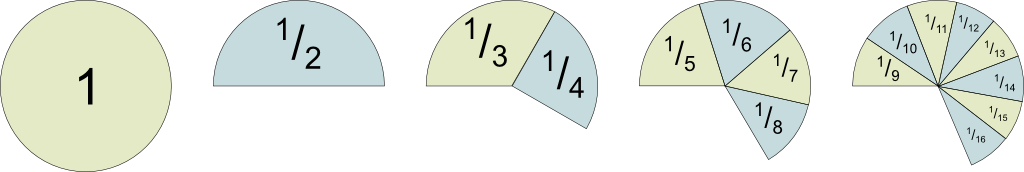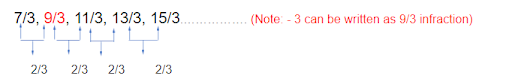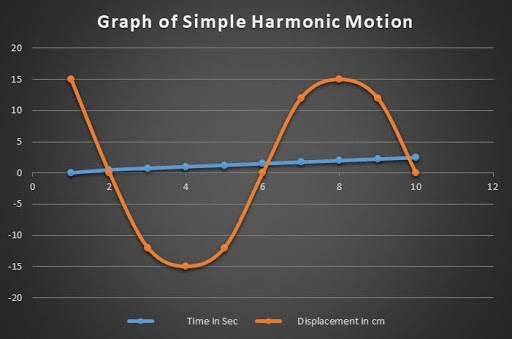Mathematics
Harmonic Sequence
0

 1 Introduction 2 Applications of Harmonic Progression in real life 3 First term of hp, Common Difference 4 nth Term Formula 5 Harmonic Sequence Formula 6 Sum of Harmonic Sequence 7 Harmonic Graph and Properties 8 Summary

23rd September 2020

## Introduction

Before we understand Harmonic Sequence or Harmonic Series, we must understand what is Arithmetic sequence /Arithmetic Progression.

I assume you all have already covered Arithmetic Progression under Sequence and Series.Here we will understand every concept of Harmonic Series following the Arithmetic sequence.

What is a harmonic series?

The reciprocal form of the Arithmetic Sequence with numbers that can never be 0 is called Harmonic Sequence. And the sum of such a sequence is known as Harmonic Series

 Example

If we have Arithmetic Sequence as 4,6,8,10,12 with the common difference of 2

i.e. d =2

The Harmonic Sequence of the above Arithmetic Sequence is

1/4, 1/6, 1/8,1/10,1/12….

Let’s take another example

We have to determine if the below series is Harmonic series or not

3/7,1/3,3/11,3/13,3/15….

Now if we prove that the reciprocal of the above sequence is A.P with a common difference then we can establish that the sequence is the Harmonic sequence . And the sum of this sequence would be a harmonic series.

So, the A.P of the above sequence can be expressed asSo, the difference between the 1st and 2nd fraction in the sequence is 2/3 and the same is the case with 2nd and 3rd fraction or 3rd and the 4th.

Note:

Trick here is whenever you are given Harmonic Progression convert it into A.P

 Example:

Find the next four terms of the sequence: - 1/7, -1/2, -1/11 ….

d = -2-7 = -9

So, the NEXT Sequence will be

x- (-11) = -9,

-x+11 = -9   or x = -20

So, we can write

-1/11+ (-9) = -1/20

-1/20 + (-9) = -1/29

-1/29 + (-9) = -1/38

-1/38 + (-9) = -1/47

 Therefore, next four terms in the sequence is 1/7, -1/2, -1/11, -1/20, -1/29, 1/38, -1/47

## Applications of Harmonic Progression in real life

Learning about pattern and sequence is not just very important in maths but real life too. Can you imagine watching a film without a plot or series of related events and just see random scenes? So, the script writer has to ensure a series of related events or sequences of scenes in which the film would be created so that it makes sense to the audience.

The harmonic formulae can also be used by scientists to conclude the value of their experiments. For example, to establish the degree at which water boils each time the temperature is changed with the same value.

It is also used in the music industry to establish theories on sounds and to closely study them.The concept of Harmonics is also used in electrical gadgets or electrical machines and generation of power.## First term of hp, Common Difference

First-term of Harmonic Progression

The first term of the Harmonic progression is fundamental to the number series which is denoted as a. The sum of the series can never be an integer except for the first term as 1.

Common Difference

The common difference here would mean that the difference between any two-consecutive number in the series would be the same. This common difference is denoted as d.

 Example 1

If 1/a, 1/b, 1/c are three quantities in Harmonic Progression then we can say here first term is 1/a and common difference

d = 1/a -1/b= 1/c -1/b

Or a-b /ab = b-c/bc

Or a/c = b-c / a-b

## nth Term Formula

The nth term of H.P = 1/ (nth term of corresponding A.P.)

Now how do we establish this?

Let’s say in A. P the 1st term is a, 2nd term is a1, 3rd term is a3……. then the nth term is an

Or We can say recursive formulae of the Arithmetic sequence is

a+(1-1) d, a+(2-1) d, a+(3-1) d……… a+(n-1) d

Therefore, the generic Arithmetic Sequence nth term formula is

\begin{align}{a_n} = a + (n - 1) \times d\end{align}

## Harmonic Sequence Formula

Similarly

In Harmonic Progression the first term from the above expression would be

1/a, 2nd term is 1/a1, 3rd term is 1/a3……. then the nth term is 1/an

Then the recursive formula of Harmonic Sequence would be

1/ [a+(1-1) d], 1/[a+(2-1) d,] 1/[a+(3-1) d] ……… 1/[a+(n-1) d]

Note: - Recursive means pattern repetitive in nature so to find out the next term we should look at the previous term and add the common difference of the series. Also, that the first term should be given to us.

Therefore, we can say

The Harmonic Sequence formulae are nth term or the general term of H.P

\begin{align}{a_n} = \frac{1}{{a + (n - 1)d}}\end{align}

 Example 1

Find out the 10th term of the below Harmonic Progression

2,2/3,2/5….

Now A.P here is 1/2,3/2,5/2

First term a = 1/2

n = 10

Therefore d= 1Hence placing the above numbers in Harmonic generic term formulae an =   we get

a10 = 1/ [1/2+(10-1) *1]
= 1/ (19/2)

 10th term of the above H.P is 2/19

## Sum of Harmonic Sequence

For an HP, the Sum of the harmonic sequence can be easily calculated if the first term and the total terms are known.

Sum of first n terms = 1/a + 1/(a + d) + 1/(a + 2d) + … +1/ [a + (n – 1) × d]

Note:- Here we can also say n refers to infinity ∞

Then the generic formulae for nth term of Harmonic sequence is the reciprocal of A.P

(as mentioned above) Similarly

the sum of ‘n’ terms of AP is Sn = n/2[2a + (n − 1) × d]

The sum of ‘n’ terms of HP is the reciprocal of A.P i.e.

\begin{align}{S_n} = \frac{2}{n}[2a + (n - 1) \times d]\end{align}

 Example 1

Find the sum of the below Harmonic Sequence.

1/12 + 1/24 + 1/36 +1/48 +1/60

Here A.P is 12,24,36,48,60

a= 12, d=12, n=5

Placing the above values in the sum of A.P formula is

\begin{align}{S_5} &= \frac{5}{2}[2 \times 12 + (5 - 1) \times 12]\\ &= [24 + 48]\frac{5}{2}\\ &= 180\end{align}

 Therefore the sum of 5 terms of H.P is reciprocal of A.P is 1/180

## Harmonic Graph and Properties

Harmonic graphs mathematical or logical models to plot harmonic motions or harmonic series.

Let’s take the example of the pendulum in which we will measure oscillation that measures different positions of the pendulum and the time it takes to reach these positions.

When we pull the pendulum to the right so that it displaces 15 cm and then release so that it oscillates that is moving back and forth.

When we pull the pendulum to the right, the position of the pendulum before we release it is 15 cm at Time (t) =0.

We will notice that it takes 2 seconds to reach the maximum height of -15cm towards the left side of the equilibrium point.

It takes another 1 second to reach back to the position it started. Also notice that the position of the pendulum, anything on the right from its original position i.e. 0 cm is positive and anything on the left is negative.

To understand this further let’s capture some more position/displacement of the pendulum at a different time of its oscillation.

Time in Sec Displacement in cm
0 15
0.5 0
0.75 -12
1 -15
1.25 -12
1.50 0
1.75 12
2.00 15
2.25 12
2.50 0

Now we plot the above table on a graph we get the below where the X-axis is the displacement (d) and Y-axis is the Time (t).We can see in the above graph that the curve is the sine curve and this is what we get for any simple harmonic motion. For example, even if we use a spring with a weight to oscillate, we will get the same harmonic graph of such a harmonic series as above.

## Summary

You will be surprised to know that the study of the Harmonic sequence dates back to the 6th century when the Greek mathematician Pythagoras studied the nature of the universe. He first used it for the study of music. Harmonic series is an infinite series that does not have any limit where the sum of successive terms tends to infinity.